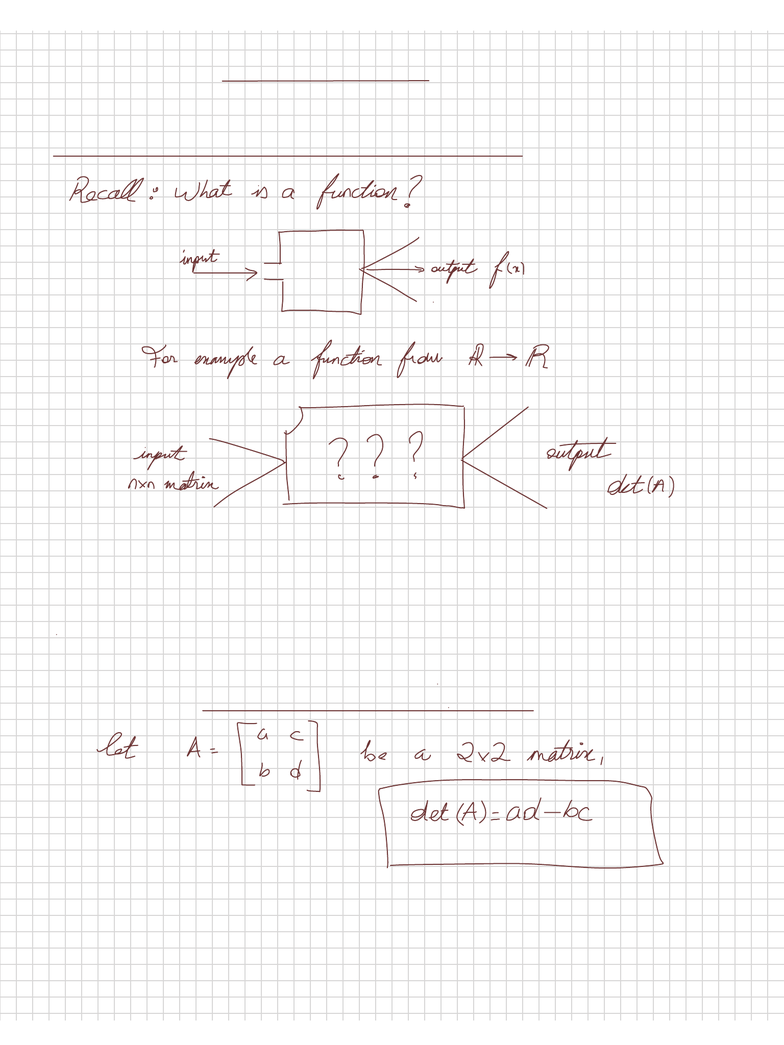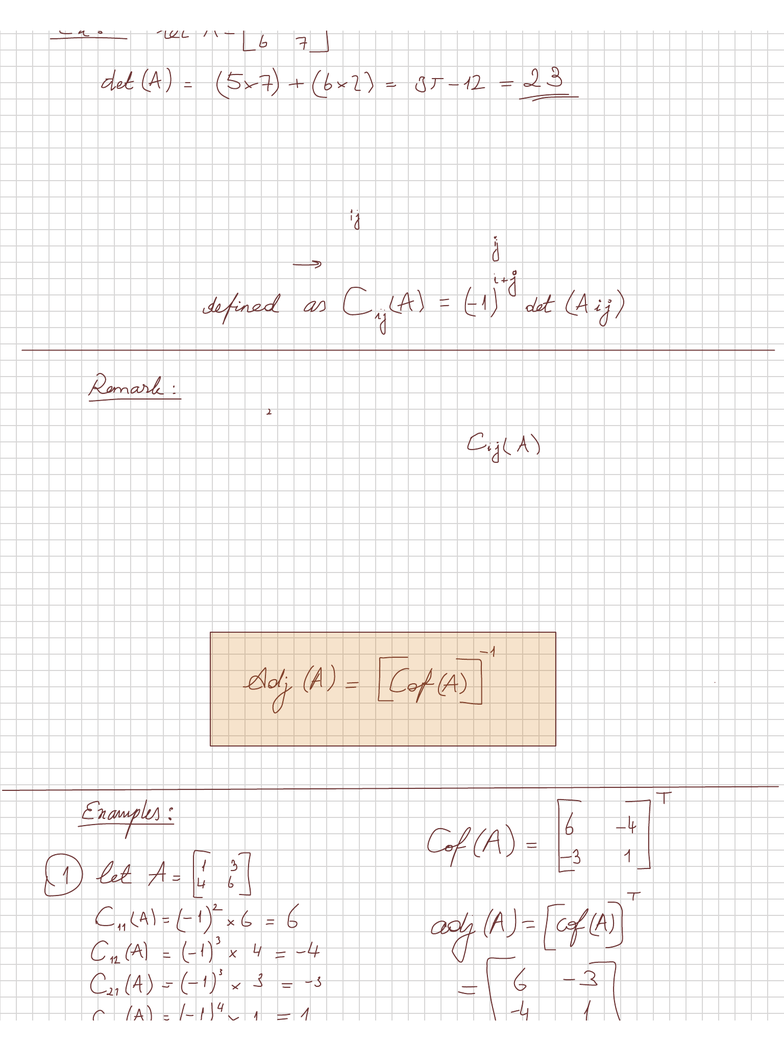Class Notes (1,100,000)
CA (650,000)
McGill (40,000)
MATH (200)
MATH 133 (300)
Lecture

# MATH 133 Lecture Notes - Laplace Expansion

Department
Mathematics & Statistics (Sci)
Course Code
MATH 133
Professor
Djivede Kelome

This preview shows pages 1-2. to view the full 6 pages of the document.Determinants
Deﬁnitions cofactor expansion (refer to 2.1)
We can view det (read determinant) as a function which uses a square
matrix(ie a nxn matrix) as a input and the output is a real number denoted
det(A)
Determinant of a 2x2 matrix

Only pages 1-2 are available for preview. Some parts have been intentionally blurred.Deﬁnition: cofactor
Let A be a nxn matrix let A. be the sub matrix of A obtained by
deleting the ith row and the jth column of A. Note that A. Is an !
(n-1)x(n-1) matrix
Since i and j can take any value from 1 to n there
are n Cofactors. We can therefore deﬁne a matrix
such that its (i,j) entry is
such matrix is called cofactor matrix (of A) we will
donote it Cof(A)
Deﬁnition:
Adjoint of A: Given A a nxn matrix, the adjoint of A is
the nxn matrix deﬁned as